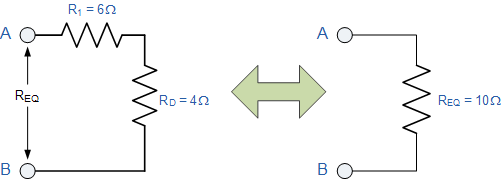## 串联电阻和并联电阻No2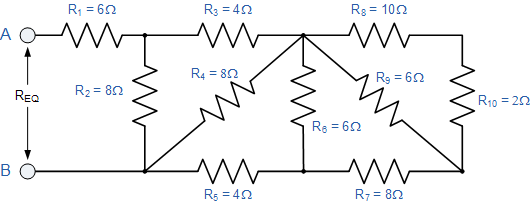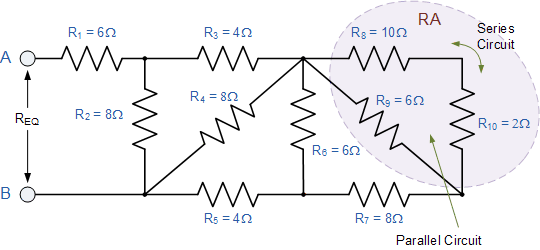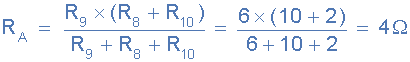R A与R 7串联，因此总电阻为R A + R 7 = 4 + 8 =12Ω，如图所示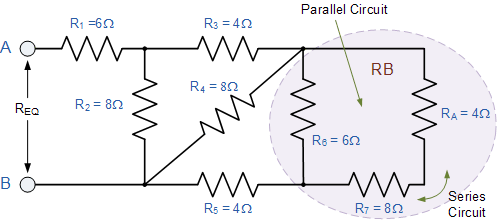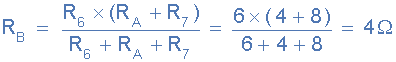R B与R 5串联，因此总电阻为R B + R 5 = 4 + 4 =8Ω，如图所示。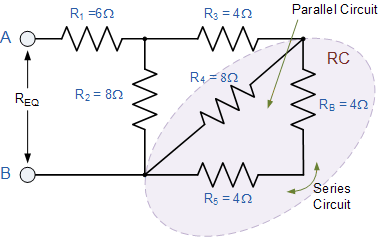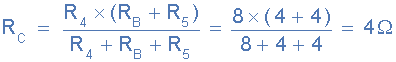R C与R 3串联，因此总电阻为R C + R 3 =8Ω，如图所示。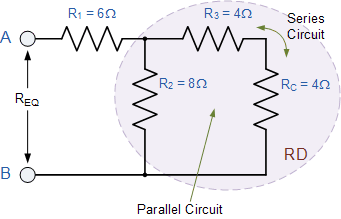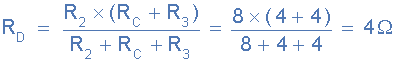R D与R 1串联，因此总电阻为R D + R 1 = 4 + 6 =10Ω，如图所示。